• 按照上篇博文所画出来的频谱图中，原信号的每个频率是准确地找出来了，但是各个频率点所对应的的幅值可不是原信号中真正的幅值，因为在进行DFT(FFT)变换的时候，已经把幅值改变了，要想让频谱图的纵坐标显示为原信号...
按照上篇博文所画出来的频谱图中，原信号的每个频率是准确地找出来了，但是各个频率点所对应的的幅值可不是原信号中真正的幅值，因为在进行DFT(FFT)变换的时候，已经把幅值改变了，要想让频谱图的纵坐标显示为原信号中真正的幅值其实也很简单，代码如下：
clear all
fs=150;%采样频率要大于等于原信号中最高频率的二倍
N=150;
t=(0:N-1)/fs;
y=0.5*sin(2*pi*65*t)+0.8*cos(2*pi*40*t)+0.7*cos(2*pi*30*t);
F=fft(y,N);
freq=(0:N/2)*fs/N;%只画(0,fs/2)范围内的频率分量，起始坐标是0，因为只有(0,fs/2)范围内的频率才有意义，因为原信号的最大频率小于等于fs
F1=abs(F(1:N/2+1));%频谱图中各频率点对应的幅值并不是原信号中真正的幅值
F2=abs(F(1:N/2+1))*2/N;%频谱图中各频率点对应的的幅值就是原信号中真正的幅值
subplot(211)
stem(freq,F1,'k');%依据频率坐标来绘制傅里叶变换后的信号的频率-幅度谱，注意F的起始坐标是1
xlabel('频率(Hz)');
ylabel('幅值');
xlim([0 130]);
subplot(212)
stem(freq,F2,'k');
xlabel('频率(Hz)');
ylabel('幅值');
xlim([0 130]);

运行结果：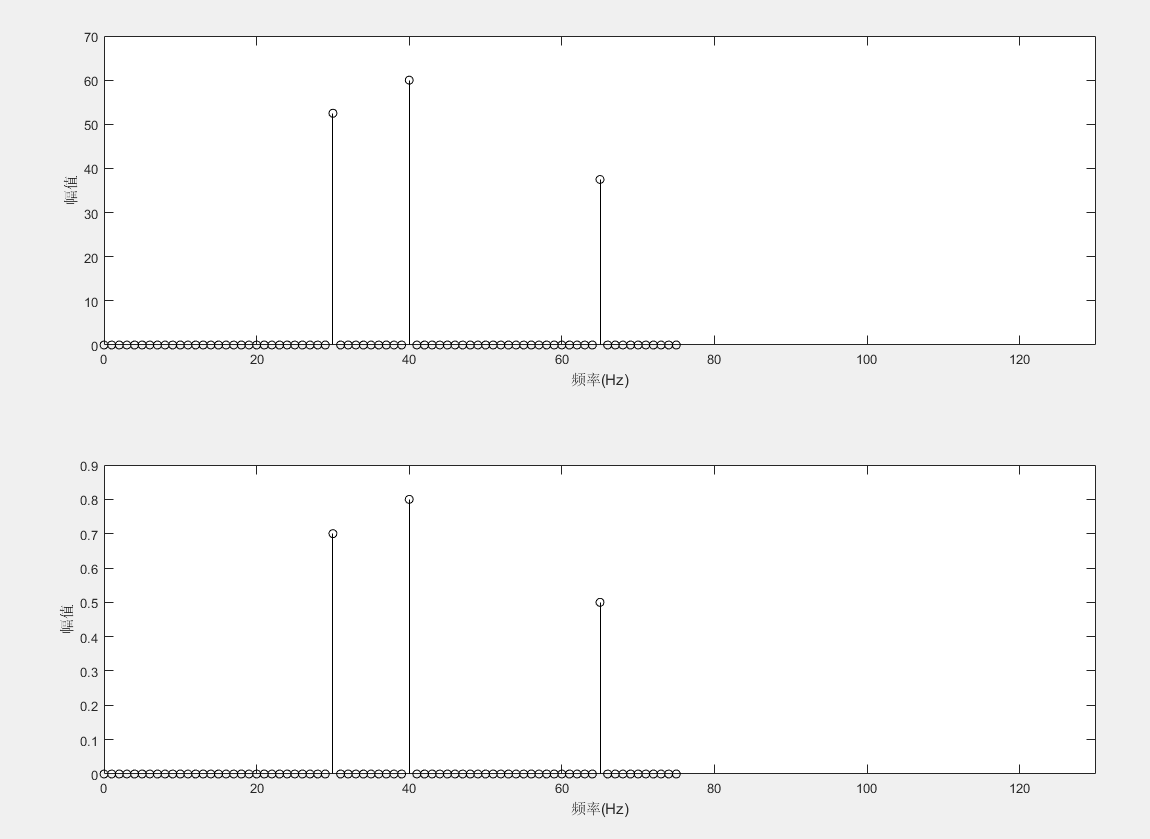我们只需要把之前的语句F1=abs(F(1:N/2+1));
更改为：F2=abs(F(1:N/2+1))*2/N; 即可，也就是说FFT变换后的各频率点对应的幅度和原信号真正的幅度就相差了一个2/N或者说是N/2的关系。
接下来再看一个例子：
clear all
fs=15000;%采样频率要大于等于原信号中最高频率的二倍
N=15000;
t=(0:N-1)/fs;
y=0.5*sin(2*pi*1000*t)+0.8*cos(2*pi*3000*t)+0.7*cos(2*pi*6000*t);
F=fft(y,N);
freq=(0:N/2)*fs/N;%只画(0,fs/2)范围内的频率分量，起始坐标是0，因为只有(0,fs/2)范围内的频率才有意义，因为原信号的最大频率小于等于fs
F1=abs(F(1:N/2+1));%频谱图中各频率点对应的幅值并不是原信号中真正的幅值
F2=abs(F(1:N/2+1))*2/N;%频谱图中各频率点对应的的幅值就是原信号中真正的幅值
subplot(211)
plot(freq,F1,'k');%依据频率坐标来绘制傅里叶变换后的信号的频率-幅度谱，注意F的起始坐标是1
xlabel('频率(Hz)');
ylabel('幅值');
xlim([0 8000]);
subplot(212)
plot(freq,F2,'k');
xlabel('频率(Hz)');
ylabel('幅值');
xlim([0 8000]);
ylim([0 1]);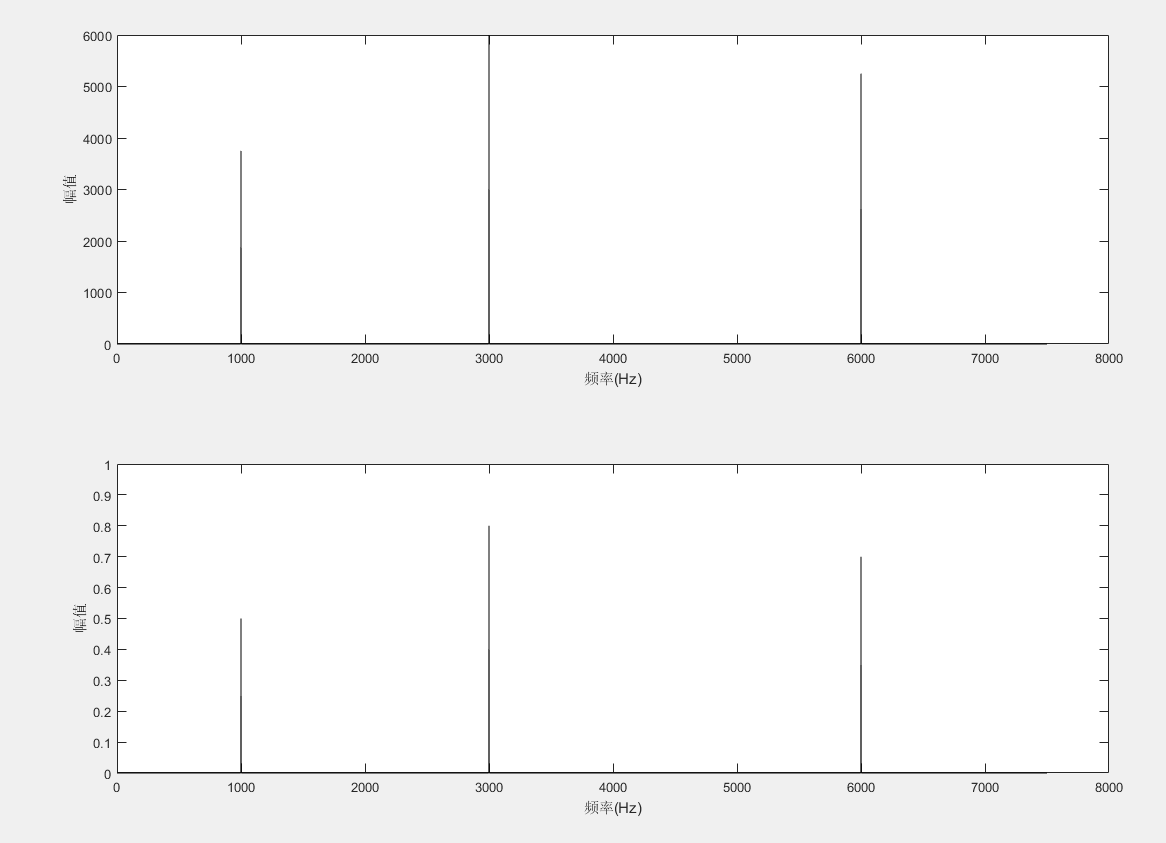展开全文• Matlab绘制短时傅里叶变换的频谱图和时间-频率-幅值三维图 function [t,frequency,f_spectrum]=fft_s(y,windowlength,Fs) % 输入 : % y-输入信号 % windowlength-窗长度 0-1的系数，比如windowlength-0.5；采样...
Matlab绘制短时傅里叶变换的频谱图和时间-频率-幅值三维图

function [t,frequency,f_spectrum]=fft_s(y,windowlength,Fs)
% 输入 :
% y-输入信号
% windowlength-窗长度 0-1的系数，比如windowlength-0.5；采样频率1000，则窗长度为500
% Fs-采样频率
windowlength = windowlength*Fs;
y = reshape(y,windowlength,[]); % 数据分段

s = fft(y); % 快速傅里叶变换

d = abs(s(1:windowlength/2,:));% 求绝对值
f_spectrum = 2/windowlength*d; % 求幅值

t = (1:size(y,2))*windowlength/Fs; % 计算时间
frequency = (0:windowlength/2-1)/windowlength*Fs; % 频率范围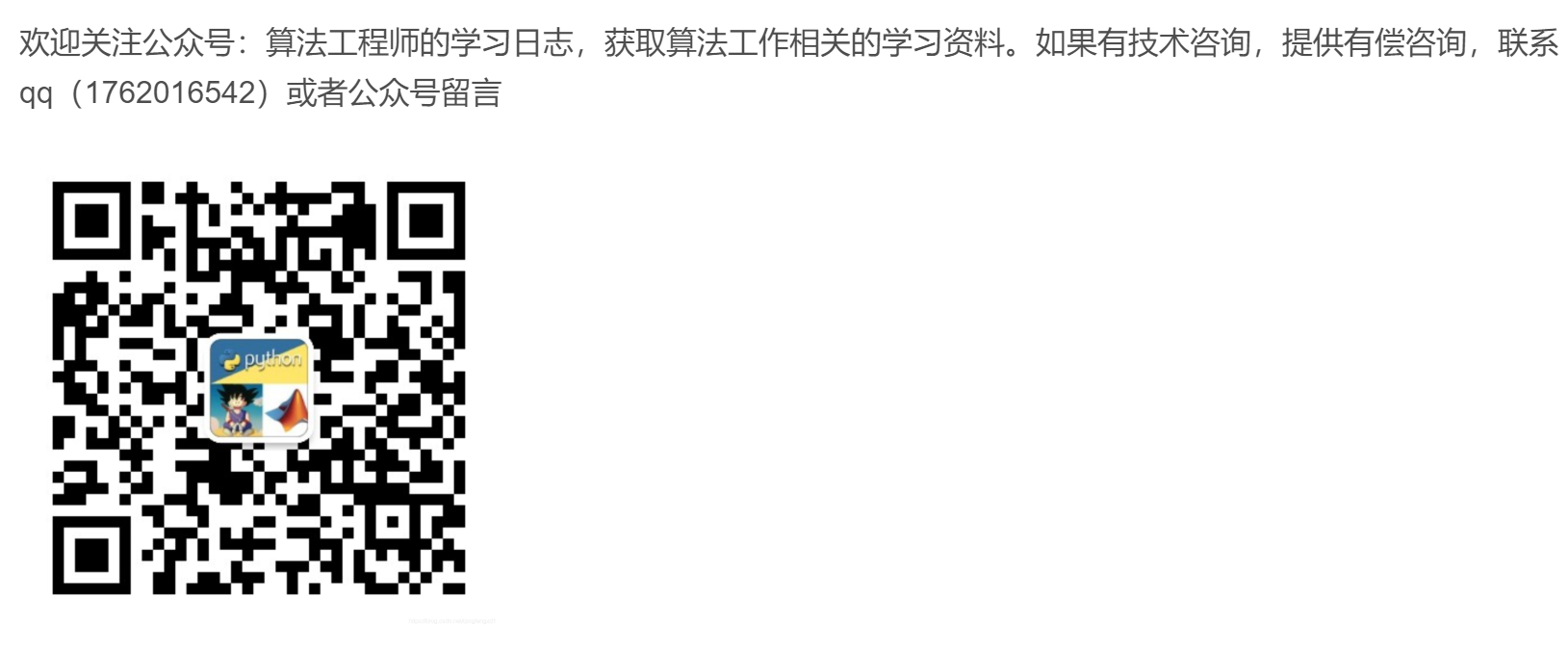展开全文Matlab 短时傅里叶变换
• 频谱图：表现的是一段音频在某一时刻各个频率的音量的高低，其横轴是频率方向，纵轴为振幅方向。 将复合波形进行傅里叶变换，拆解还原成每个频率上单一的正弦波构成，相当于把二维的波形图往纸面方向拉伸，变成了三...
文章目录时域和频域1. 概述2.（时域）波形和频域：用几张对比图来区分2.1 时域和频域2.2 区分：时频谱图（语谱图）
傅里叶变换的典型用途是将信号分解成频率谱——显示与频率对应的幅值大小。
时域和频域
1. 概述
（1）什么是信号的时域和频域？
时域和频域是信号的基本性质，用来分析信号的不同角度称为域，一般来说，时域的表示较为形象与直观，频域分析则更为简练，剖析问题更为深刻和方便。目前，信号分析的趋势是从时域向频域发展。然而，它们是互相联系，缺一不可，相辅相成的。
（2）时频域的关系是什么？
时域分析与频域分析是对模拟信号的两个观察面。对信号进行时域分析时，有时一些信号的时域参数相同，但并不能说明信号就完全相同。因为信号不仅随时间变化，还与频率、相位等信息有关，这就需要进一步分析信号的频率结构，并在频率域中对信号进行描述。动态信号从时间域变换到频率域主要通过傅立叶级数和傅立叶变换实现。周期信号的变换采用傅立叶级数，非周期信号的变换采用傅立叶变换。
（3）信号的时域和频域表达方式各有什么特点？
我们描述信号的方式有时域和频域两种方式，时域是描述数学函数或物理信号对时间的关系，而频域是描述信号在频率方面特性时用到的一种坐标系，简单来说，横坐标一个是时间，一个是频率。
一般正弦信号可由幅值、频率、相位三个基本特征值就可以唯一确定。但对于两个形状相似的非正弦波形，从时域角度，很难看出两个信号之间的本质区别，这就需要用到频域表达方式。
小结

时域：自变量是时间，即横轴是时间，纵轴是信号的变化（振幅）。其动态信号$x(t)$是描述信号在不同时刻取值的函数。
频域：自变量是频率，即横轴是频率，纵轴是该频率信号的幅度（振幅），就是指的信号电压大小，也就是通常说的频谱图。

2.（时域）波形和频域：用几张对比图来区分
2.1 时域和频域

时域 vs 频域时域波形、频域谱线时域图：表现的是一段音频在一段时间内音量的变化，其横轴是时间方向，纵轴是振幅方向。
波形实质上是将各个频率的波形叠加在了一起（波形是由各频率不同幅值和相位的简单正弦波复合叠加得到的。）
频谱图：表现的是一段音频在某一时刻各个频率的音量的高低，其横轴是频率方向，纵轴为振幅方向。
将复合波形进行傅里叶变换，拆解还原成每个频率上单一的正弦波构成，相当于把二维的波形图往纸面方向拉伸，变成了三维的立体模型，而拉伸方向上的那根轴叫频率，现在从小到大每个频率点上都对应着一条不同幅值和相位的正弦波。
频谱则是在这个立体模型的频率轴方向上进行切片，丢去时间轴（即在每个时刻都可以拿刀在与时间轴垂直的方向上进行切片），形成以横坐标为频率，纵坐标为幅值的频谱图，表示的是一个静态的时间点上各频率正弦波的幅值大小的分布状况。
再说的直白一点，频谱就是为了找出一个波是由多少波复合而成的！
关于为什么是正弦波，可以查看之前的文章：从本质（信号分析角度）理解卷积
从下面的频谱图中可以得出这样的结论：

原始波由三个正弦波叠加而成；
横轴为这些正弦波分量的频率，纵轴为这些正弦波分量的振幅。2.2 区分：时频谱图（语谱图）
语谱图：先将语音信号作傅里叶变换，然后以横轴为时间，纵轴为频率，用颜色表示幅值即可绘制出语谱图。在一幅图中表示信号的频率、幅度随时间的变化，故也称“时频图”。如下面两张图分别为数字0-10的波形图和语谱图：

（1）数字0-10的波形图：（2）数字0-10的语谱图：附：

频宽、带宽、频带？
频带（frequency band）：对信号而言，频带就是信号包含的最高频率与最低频率这之间的频率范围(当然频率分量必须大于一定的值)。对信道而言，频带就是允许传送的信号的最高频率与允许传送的信号的最低频率这之间的频率范围(当然要考虑衰减必须在一定范围内)
频带宽度（band width）：简称带宽，有时称必要宽度，指为保证某种发射信息的速率和质量所需占用的频带宽度容许值，以赫（Hz）、千赫（KHz）、兆赫（MHz）表示。
注意区分：网络带宽，是指在单位时间能传输的数据量，亦即数据传输率。
宽带和窄带？
“窄”和“宽”是一个相对概念，并无严格数字界限，相对于什么呢？是指信道特性相对于信号特性。第一，什么叫宽带信号，“有待传输的信号”我们称为信源，信源是具备一定的频谱特征的。信源信号通常需要一个载波信号来调制它，才能发送到远方。信源信号带宽远小于载波中心频率的是窄带信号,反之，二者大小可比拟的称为宽带信号。
第二，实际通信中，分配给你的频带资源+真实的传播环境, 我们称之为信道。信道也具备一定的频谱特征。通常情况下，分配到的频带资源越宽，传播环境越稳定，信道能够承载的数据速率就越高。

更多参考：音色与声谱图——很详细的介绍
参考：

如何理解 图像傅里叶变换的频谱图

频域（频谱）图和时域图横纵坐标及分析

声音的波形和频谱是什么？它们两者有什么联系？

语音信号语谱图分析

什么是信号的时域和频域？——比较精练的总结

信号频域分析方法的理解（频谱、能量谱、功率谱、倒频谱、小波分析） - Mr.括号的文章 - 知乎——比较全面的介绍

Matlab 语谱图(时频图)绘制与分析


展开全文• 先直接调用FFT函数实现频谱分析 y = fft(x,N); Fs = 5120; dt = 1.0/Fs; N = 1024; T = dt * N; t = linspace(0,T,N); x = 10*sin(2*pi*100*t)+5*sin(2*pi*300*t)+2*sin(2*pi*700*t); plot(t,x); y = fft(x,N); a =...

先直接调用FFT函数实现频谱分析 y = fft(x,N);

Fs = 5120;
dt = 1.0/Fs;
N = 1024;
T = dt * N;
t = linspace(0,T,N);
x = 10*sin(2*pi*100*t)+5*sin(2*pi*300*t)+2*sin(2*pi*700*t);
plot(t,x);
y = fft(x,N);
a = real(y);
b = imag(y);
figure
subplot(211)
plot(a);
subplot(212)
plot(b);
A1 = abs(y);
Q1 = angle(y)*180/pi;
figure
subplot(211)
plot(A1);
subplot(212)
plot(Q1)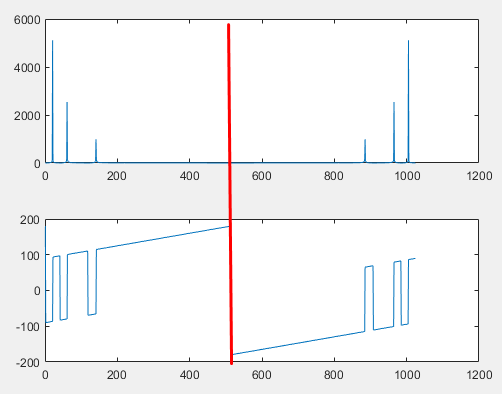调用FFT结果中含有负频率部分，有用数据只有512个。1024个点的计算结果只要显示前512个点，后面是负频率的镜像映射，没有意义

f = linspace(0,Fs/2,N/2); % 消除负频率


整理后代码如下

Fs = 5120;
dt = 1.0/Fs;
N = 1024;
T = dt * N;
t = linspace(0,T,N);
x = 10*sin(2*pi*100*t)+5*sin(2*pi*300*t)+2*sin(2*pi*700*t);
plot(t,x);
y = fft(x,N);
f = linspace(0,Fs/2,N/2); % 消除负频率
A1 = abs(y)/(N/2); % 还原幅值
Q1 = angle(y)*180/pi;
subplot(211)
plot(f,A1(1:N/2));
subplot(212)
plot(f,Q1(1:N/2))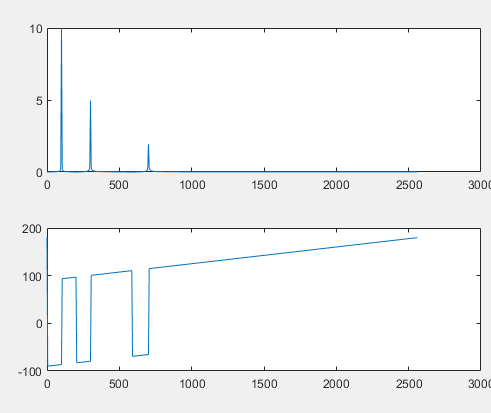相位还可以接缠绕处理 unwrap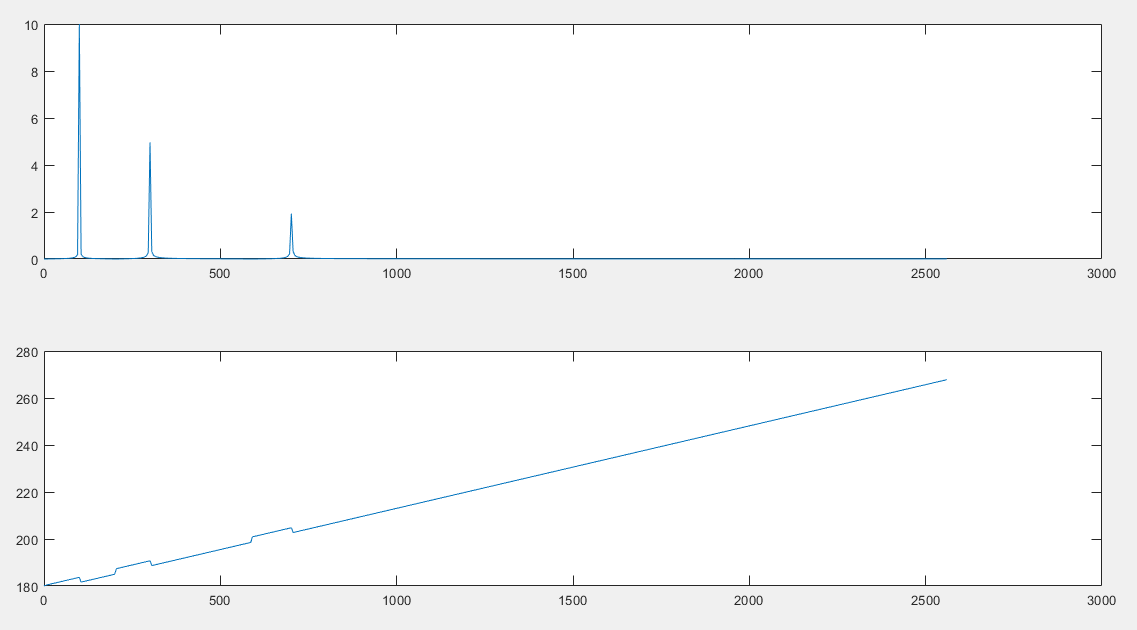对数功率谱  dB = 20log(P) 分贝
功率谱取平方，大数据和小数据之间差异很大，对数里大数据和小数据之间差距缩小了，工程上经常使用

Fs = 5120;
dt = 1.0/Fs;
N = 1024;
T = dt * N;
t = linspace(0,T,N);
x = 10*sin(2*pi*100*t)+5*sin(2*pi*300*t)+2*sin(2*pi*700*t);
plot(t,x);
y = fft(x,N);
f = linspace(0,Fs/2,N/2); % 消除负频率
A1 = abs(y)/(N/2); % 还原幅值
Q1 = unwrap(angle(y)*180/pi);
subplot(211)
plot(f,A1(1:N/2));
subplot(212)
plot(f,Q1(1:N/2))
figure % 以下计算功率谱和对数功率谱
A2 = A1.^2;
P2 = 20*log10(A2);
subplot(211)
plot(f,A2(1:N/2));
subplot(212)
plot(f,P2(1:N/2));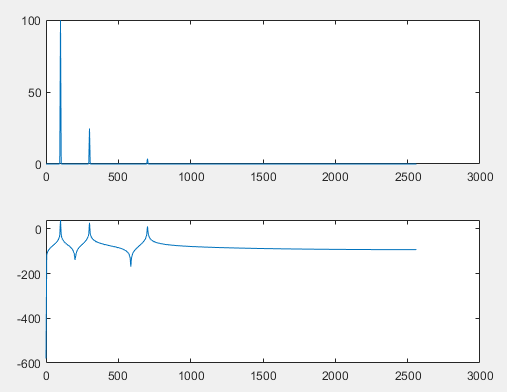方波信号看得更真实

Fs = 5120;
dt = 1.0/Fs;
N = 1024;
T = dt * N;
t = linspace(0,T,N);
x = square(100*t);
figure
subplot(311)
plot(t,x);
y = fft(x,N);
f = linspace(0,Fs/2,N/2); % 消除负频率
A1 = abs(y)/(N/2); % 还原幅值
A2 = A1.^2;
P2 = 20*log10(A2);
subplot(312)
plot(f,A2(1:N/2));
subplot(313)
plot(f,P2(1:N/2));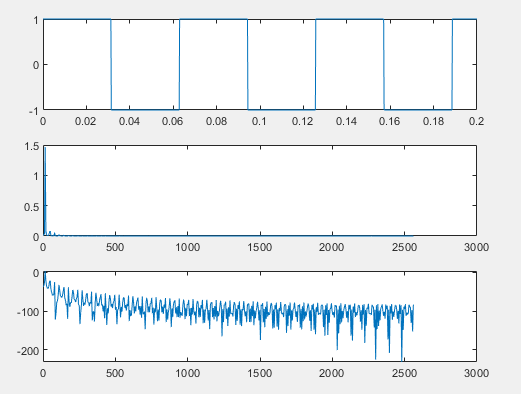代码来自华中科技大学的《数字信号分析理论与实践》，仅作学习总结


展开全文• 文章目录前言一、lena图片的傅里叶(反)变换二、令相位为0进行傅里叶反变换三、令振幅为1进行傅里叶反变换四、双谱重构五、...频谱图：频域和幅值图像&频域和相位图像统称频谱图 一、lena图片的傅里叶(反)变换 imA =图像处理
• 图像的二维傅里叶变换频谱图特点研究 一、先放一些相关的结论： 1、傅里叶变换的幅值称为傅里叶谱或频谱。 2、F(u)的零值位置与“盒状”函数的宽度W成反比。 3、卷积定理：空间域两个函数的卷积的傅里叶变换等于...
• 1、傅里叶变换的幅值称为傅里叶谱或频谱。 2、F(u)的零值位置与“盒状”函数的宽度W成反比。 3、卷积定理：空间域两个函数的卷积的傅里叶变换等于两个函数的傅里叶变换在频率域中的乘积。f(t)*h(t) <=> H(u)F...
•  但是，我们用matlab求出来的频谱图却不是这样的；原因是： 1.理论中的正弦信号是无限长连续信号，而matlab，参与运算的信号只是截取了其中1个周期或多个周期的信号，就变成 了有限长信号了；无限长信号和有限长...
• 希尔伯特黄变换的运行代码，EMD分解的IMF图形，HHT变换的时频谱图，边际谱图，顺势频率包络图，瞬时幅值包络图希尔伯特黄变换
• 数字信号频谱示意三种类型数字信号频谱示意图频谱示意绘制注意问题 不显示负频率部分 X坐标换为频率 幅值量纲还原 详细代码与解释Fs=5120;%时域采样频率 N=1024;%采样点数 dt=1.0/Fs;%时域采样时间间隔信号处理 matlab
• <p>2、直接用A的实部值作为像素值生成频谱图 但是我发现生成的频谱图布局不对。 用幅度值作为像素值生成的频谱图 <p><img alt="" src="https://img-ask.csdnimg.cn/upload/1616129352355.png" /></p> <p><img ...人工智能 机器学习 深度学习
• 幅值计算 (cv_abs)， 频谱平移 (cv_gray_fft2shift)【频谱平移的作用详见我的博文 http://blog.csdn.net/wenhao_ir/article/details/51689960 】，将 数值归一化到0到255区间 (cv_range_0to255)是非常常用的四个功能...
• 今天主要讲一下在STM32中如何利用dsp库进行快速傅里叶计算，从而得出信号的频谱幅值以及相位。 一、Matlab简单搭建 1.谐波检测搭建 通过50Hz正弦波叠加100Hz、200Hz的正弦波得到叠加后畸变的波形，这个波形主要用来...
• ## 频谱

2021-05-08 11:03:26
下面是一个简单的频谱图，其中横坐标是频率，纵坐标是振幅 可以看到在大约在300hz、800hz、1100hz、1700hz处，振幅有最大值。 如果是电信号来说，也就是在这四处频率时，幅值（一般用电压或电流表征电信号大小）较...
• disp_hhs(E,tt1) %二维显示HHT时频谱，E是求得的HHT谱 pause figure(4) for i=1:size(c,1) faa=fa(i,:); [FA,TT1]=meshgrid(faa,tt1);%三维显示HHT时频 surf(FA,TT1,E) title('HHT时频谱三维显示') hold on ...图片
• 在二维频谱分析之前，可以先分析一维DFT，由于一维的频谱很常见，横坐标u代表频率，纵坐标代表幅值，由此可知，u=0时也就是越靠近纵轴，频率越低， 二维 和一维一样做处理，在变化之后的右图，可以发现低频和...快速傅立叶变换
• 本人从事射频系统测试有一段时间了，经过工程测试实践，总结了一下的使用。可以用来测量和显示被测信号得频率和幅值，可以将复杂信号分离或...　图5、频谱图　2、dBm，dB　dB是一个比值取log是一个相对量，例如：　dB
• PC 声卡、MP3 播放器）输出端的模拟电压信号时，将其转化成一串数字信号，然后通过FFT（快速傅氏变换）运算，得出声音信号的频率分布关系，这个也就是我们常说的频谱图，通过一台虚拟示波器采集了一首歌某个时刻的...
• FFT变换的幅值和能量校正 对于从时域通过傅立叶变换计算频谱的大多数人来说，幅值和能量校正一直是个困惑点。首先要说的是，数据中包括的信息和...如果你有一个有效值为1V的正弦波（如1所示），用FFT频谱分析仪进...FFT
• 用Matlab语言产生一个信号，该信号由三个不同频率、幅值也不同且初始相位不全为零的正弦信号组成，对该信号进行时域波形分析(峰值，峰...打印编程代码及注释，同时将时域分析结果、频域的频谱图、相关图打印贴在下面。
• ## 什么是频谱

万次阅读 2017-10-17 15:17:11
频谱：就是频率的分布曲线，复杂振荡分解为振幅不同和频率不同的谐振荡，这些谐振荡的幅值按频率排列的的图形叫做频谱。 注：信号频谱的概念既包含有很强的数学理论（傅立叶变换、傅立叶级数等）；又具有明确的物理...
• ## FFT频谱学习

千次阅读 2017-01-04 21:34:59
图像的二维傅里叶变换频谱图特点研究 一、先放一些相关的结论： 1、傅里叶变换的幅值称为傅里叶谱或频谱。 2、F(u)的零值位置与“盒状”函数的宽度W成反比。 3、卷积定理：空间域两个函数的卷积的傅里叶变换...
• ## 频谱与功率谱

万次阅读 多人点赞 2019-07-03 19:31:10
复杂振荡分解为振幅不同和频率不同的谐振荡，这些谐振荡的幅值按频率排列的图形叫做频谱频谱将对信号的研究从时域引入到频域，从而带来更直观的认识。 功率谱：功率谱是功率谱密度函数的简称，是在有限信号的情况...
• 但是会影响幅值 50周期采样（左） 100周期采样（右） 100周期采样（左） 200周期采样（右） 对幅值影响已很小 1.采样步长会明显影响图像 100周期，步长1024：（左图） 100周期，步长10240：（右图） ...
• 从广义上说，信号的某种特征量随信号频率变化的关系，称为信号的频谱，所画出的图形称为信号的频谱图。 【 1. 周期信号的频谱 】 周期信号的频谱指周期信号中各次谐波幅值、相位随频率变化关系。 1. 原理 周期信号的...
• 频谱图 频谱反应的是在频域下振幅随频率的变化曲线，即不同频率下的振幅变化，其横坐标为频率，纵坐标为振幅。关于振幅，即震动的物理量达到的最大值。经傅里叶变换，可以实现由时域到频域的转换。 比如上图中lora...物联网
• 实验一 [y,fs,bit]=wavread'I do%读取音乐片段fs是采样率 size(y%求矩阵的行数和列数 y1=y,1%对信号进行分列处理 n1=length(y1%取y的长度 t1=(0:... %画出时域波形 ylabel'幅值; xlabel'时间s; title'信号波形; subp...